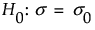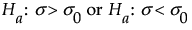Design of Experiments Guide > Prospective Sample Size and Power > One Sample Standard Deviation Calculator
Publication date: 08/13/2020

# One Sample Standard Deviation Calculator

Use the One Sample Standard Deviation calculator to evaluate sample size for a hypothesis test about one standard deviation. Sample size and power are associated with the following hypothesis test:versus the one-sided alternative:where σ is the population standard deviation and σ0 is the hypothesized value. The difference to detect is an amount, δ, away from σ0 that one considers as important to detect based on a set of samples.